Function Repository Resource:

# QuantityString

Convert a Quantity to a linear string in a customizable way

Contributed by: Sjoerd Smit
 ResourceFunction["QuantityString"][quantity] converts quantity to a linear string by abbreviating the canonical unit with standard short forms. ResourceFunction["QuantityString"][quantity,"BoxForm"] converts quantity to a linear string that resembles the default typesetting in the front end. ResourceFunction["QuantityString"][quantity, template] formats the output using a StringTemplate.

## Details

ResourceFunction["QuantityString"][quantity,"Canonical"] can also be used and does the same as ResourceFunction["QuantityString"][quantity].
The template will be filled with string versions of the TemplateBox arguments that are produced by ToBoxes[quantity].

## Examples

### Basic Examples (3)

Define a Quantity composed of different units:

 In:=Out=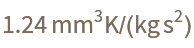Convert it to a simple String, using ^ and / to linearize the different powers:

 In:=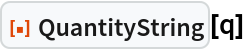Out=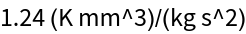Compare this with the InputForm of the QuantityUnit of this Quantity:

 In:=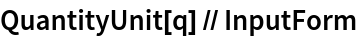Out=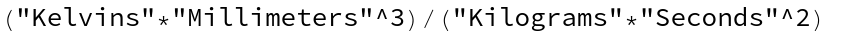### Scope (4)

Display the unit in the same way the FrontEnd does:

 In:=Out=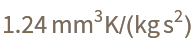In:=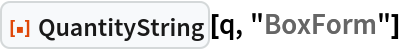Out=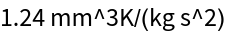In:=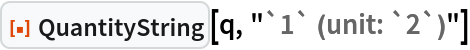Out=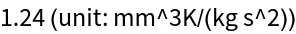See all the elements that you can access inside of the template:

 In:=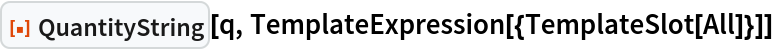Out=Use this to define a more elaborate output:

 In:=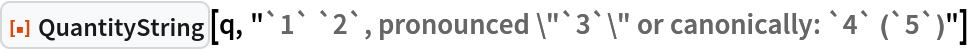Out=Not all types of quantities have only four elements:

 In:=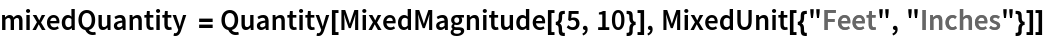Out=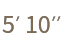In:=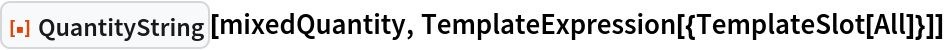Out=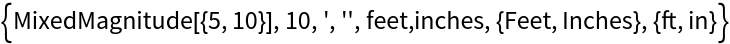Most of the elements available in the template come from the box representation of the quantity:

 In:=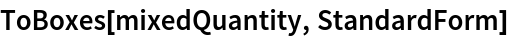Out=The "BoxForm" output replaces the superscript primes with apostrophes:

 In:=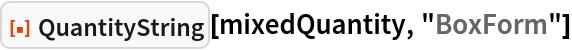Out=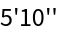The canonical form riffles the magnitudes with the canonical unit abbreviations:

 In:=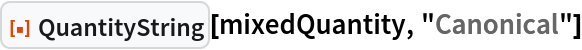Out=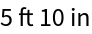Convert different currencies. Note that the position of the currency sign is respected:

 In:=Out=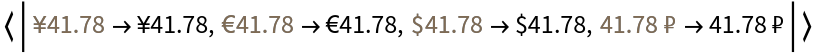Dated units are supported:

 In:=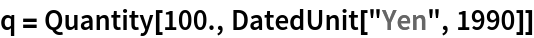Out=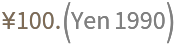In:=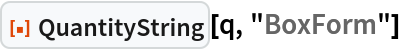Out=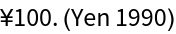In the canonical form, the date will be typeset in the ISO format:

 In:=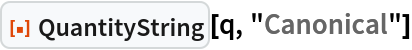Out=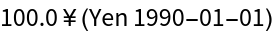### Possible Issues (2)

There is no guarantee that the strings can be converted back to quantities again:

 In:=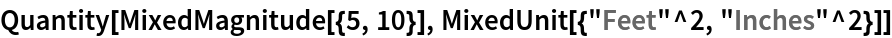Out=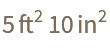In:=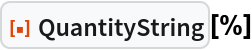Out=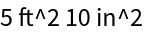In:=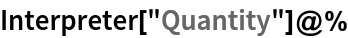Out=The canonical unit string always places the unit after the magnitude, which is not always the typical way to typeset a unit:

 In:=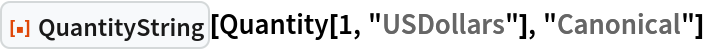Out=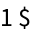Use a template or the BoxForm appearance to typeset the canonical unit differently:

 In:=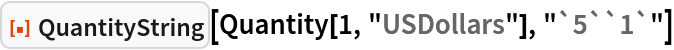Out=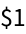In:=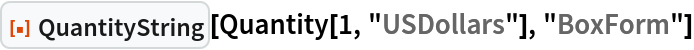Out=Sjoerd Smit

## Version History

• 1.0.0 – 06 September 2022